In :
import pymc3 as pm
import numpy as np
import pandas as pd
import seaborn as sns
import matplotlib.pyplot as plt

%matplotlib inline
%config InlineBackend.figure_format = 'retina'
%qtconsole --colors=linux
plt.style.use('ggplot')

from matplotlib import gridspec
from theano import tensor as tt
from scipy import stats


# Chapter 14 - Multinomial processing trees¶

## 14.1 Multinomial processing model of pair-clustering¶

Multinomial processing trees (MPTs: Batchelder & Riefer, 1980, 1986; Chechile, 1973; Chechile & Meyer, 1976) provide one approach to modeling the finding that semantically related items are often recalled consecutively. An MPT model assumes that observed behavior arises from a sequence of cognitive events, able to be represented by a rooted tree architecture

$$c,r,u \sim \text{Beta}(1,1)$$$$\theta_{1} = cr$$$$\theta_{2} = (1\,-\,c)u^2$$$$\theta_{3} = 2u\,(1\,-\,c)\,(1\,-\,u)$$$$\theta_{4} = c\,(1\,-\,r)\,+\,(1\,-\,c)\,(1\,-\,u)^2$$$$\mathbf k \sim \text{Multinomial}(\mathbf \theta,n)$$
In :
indiv_trial = {}
Nt = 3
kall = np.array([[45, 24, 97, 254],
[106, 41, 107, 166],
[243, 64, 65, 48]])
for trial in np.arange(Nt):
k = kall[trial, :]
n = sum(k)
with pm.Model() as model1:
c = pm.Beta('c', alpha=1, beta=1)
r = pm.Beta('r', alpha=1, beta=1)
u = pm.Beta('u', alpha=1, beta=1)

t1 = c*r
t2 = (1-c)*(u**2)
t3 = 2*u*(1-c)*(1-u)
t4 = c*(1-r)+(1-c)*(1-u)**2

kobs = pm.Multinomial("kobs", p=[t1, t2, t3, t4], n=n, observed=k)
trace = pm.sample(3e3, njobs=2, progressbar=False)

# keep trace for later analysis
indiv_trial[trial] = trace

Auto-assigning NUTS sampler...
/home/laoj/Documents/Github/pymc3/pymc3/step_methods/hmc/nuts.py:451: UserWarning: The acceptance probability in chain 1 does not match the target. It is 0.889704445797, but should be close to 0.8. Try to increase the number of tuning steps.
% (self._chain_id, mean_accept, target_accept))
Auto-assigning NUTS sampler...
Auto-assigning NUTS sampler...

In :
from scipy.stats.kde import gaussian_kde

fig = plt.figure(figsize=(15, 5))
gs = gridspec.GridSpec(1, 3)
x1=np.linspace(0, 1, 200)
plotparm=['c', 'r', 'u']
trialname=['Trial 1', 'Trial 2', 'Trial 6']
ax0 = {}
for trial in np.arange(Nt):
for ip, ii in enumerate(plotparm):
cpost = indiv_trial[trial][ii][50:]
ax0[ip] = plt.subplot(gs[ip])
my_pdf = gaussian_kde(cpost)
ax0[ip].plot(x1, my_pdf(x1), lw=2.5, alpha=0.6) # distribution function
plt.xlim([0, 1])
plt.xlabel(ii)

ax0 = plt.subplot(gs)
plt.legend(trialname, loc='upper left')
plt.ylabel('Probability Density')
plt.show()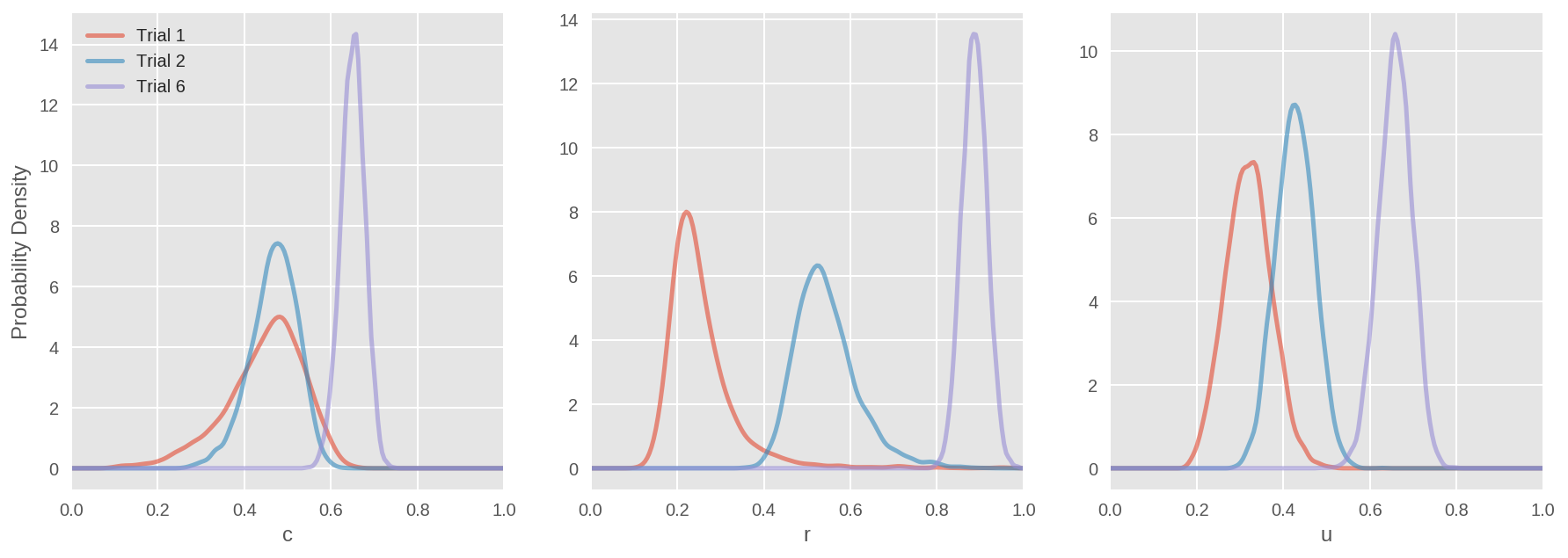## 14.2 Latent-trait MPT model¶

$$\hat \mu^c, \hat \mu^r, \hat \mu^u \sim \text{Gaussian}(0,1)$$$$\hat \xi^c, \hat \xi^r, \hat \xi^u \sim \text{Uniform}(0,100)$$$$\Sigma^{-1} \sim \text{Wishart}(I,4)$$$$(\hat \delta_{i}^c,\hat \delta_{i}^r,\hat \delta_{i}^u) \sim \text{MvGaussian}(0,\Sigma^{-1})$$$$c_{i} = \Phi(\hat \mu^c\,+\,\hat \xi^c\hat \delta_{i}^c)$$$$r_{i} = \Phi(\hat \mu^r\,+\,\hat \xi^r\hat \delta_{i}^r)$$$$u_{i} = \Phi(\hat \mu^u\,+\,\hat \xi^u\hat \delta_{i}^u)$$$$\theta_{i1} = c_i r_i$$$$\theta_{i2} = (1\,-\,c_i)u_i^2$$$$\theta_{i3} = 2u_i\,(1\,-\,c_i)\,(1\,-\,u_i)$$$$\theta_{i4} = c_i\,(1\,-\,r_i)\,+\,(1\,-\,c_i)\,(1\,-\,u_i)^2$$$$\mathbf k_i \sim \text{Multinomial}(\mathbf \theta_i,n)$$
In :
### Riefer et al (2002) data:
Nsubj = 21
Nitem = 20
response_1=np.array([2,4,4,10,2,1,3,14,2,2,5,11,6,0,4,10,1,
0,4,15,1,0,2,17,1,2,4,13,4,1,6,9,5,1,4,
10,1,0,9,10,5,0,3,12,0,1,6,13,1,5,7,7,1,
1,4,14,2,2,3,13,2,1,5,12,2,0,6,12,1,0,5,
14,2,1,8,9,3,0,2,15,1,2,3,14]).reshape(21,-1)
response_2=np.array([7,5,3,5,5,2,3,10,6,2,7,5,9,4,2,5,2,2,7,
9,1,3,3,13,5,0,5,10,7,3,4,6,7,3,6,4,4,1,
10,5,9,1,2,8,3,1,6,10,3,5,9,3,2,0,6,12,
8,0,3,9,3,2,7,8,7,1,5,7,2,1,6,11,5,3,5,
7,5,0,6,9,6,2,2,10]).reshape(21,-1)
response_6=np.array([14,3,1,2,12,3,1,4,18,0,1,1,15,3,0,2,7,
1,10,2,3,6,11,0,8,4,3,5,17,1,1,1,13,4,
3,0,11,6,1,2,16,1,2,1,10,1,3,6,7,13,0,
0,8,4,3,5,16,1,1,2,5,4,7,4,15,0,5,0,6,
3,6,5,17,2,0,1,17,1,0,2,8,3,6,3]).reshape(21,-1)
kall={}
kall = response_1
kall = response_2
kall = response_6

In :
p = 3
nu= p+2
indiv_trial2 = {}
Nt = 3

def Phi(x):
# probit transform
return 0.5 + 0.5 * pm.math.erf(x/pm.math.sqrt(2))

for trial in np.arange(Nt):
k = kall[trial]
with pm.Model():
mu = pm.Normal('mu', mu=0, sd=1, shape=p)
xi = pm.Uniform('xi', lower=0, upper=100, shape=p)

wishart = pm.WishartBartlett('wishart', S=np.eye(p), nu=nu)
cov = tt.nlinalg.matrix_inverse(wishart)

delta = pm.MvNormal('delta', mu=np.zeros(Nt), cov=cov, shape=(Nsubj, p))

c = Phi(mu+xi*tt.dot(delta, [1, 0, 0]))
r = Phi(mu+xi*tt.dot(delta, [0, 1, 0]))
u = Phi(mu+xi*tt.dot(delta, [0, 0, 1]))

t1 = c*r
t2 = (1-c)*(u**2)
t3 = 2*u*(1-c)*(1-u)
t4 = c*(1-r)+(1-c)*(1-u)**2

muc = pm.Deterministic('muc', Phi(mu))
mur = pm.Deterministic('mur', Phi(mu))
muu = pm.Deterministic('muu', Phi(mu))

#         p_ = tt.stack([t1, t2, t3, t4])
#         kobs = pm.Multinomial('kobs', p=p_, n=Nitem, observed=k.T)
# Multinomial data likelihood
kobs = [pm.Multinomial('kobs_%i'%i, p=[t1[i], t2[i], t3[i], t4[i]], n=Nitem,
observed=k[i]) for i in np.arange(Nsubj)]

trace = pm.sample(5e4, init=None, step=pm.Metropolis())

# keep trace for later analysis
indiv_trial2[trial] = trace

Added new variable c to model diagonal of Wishart.
Added new variable z to model off-diagonals of Wishart.
100%|██████████| 50500/50500.0 [03:03<00:00, 275.17it/s]
Added new variable c to model diagonal of Wishart.
Added new variable z to model off-diagonals of Wishart.
100%|██████████| 50500/50500.0 [03:12<00:00, 262.50it/s]
Added new variable c to model diagonal of Wishart.
Added new variable z to model off-diagonals of Wishart.
100%|██████████| 50500/50500.0 [02:58<00:00, 282.91it/s]

In :
for trial in np.arange(Nt):
pm.traceplot(indiv_trial2[trial], varnames=['mu']);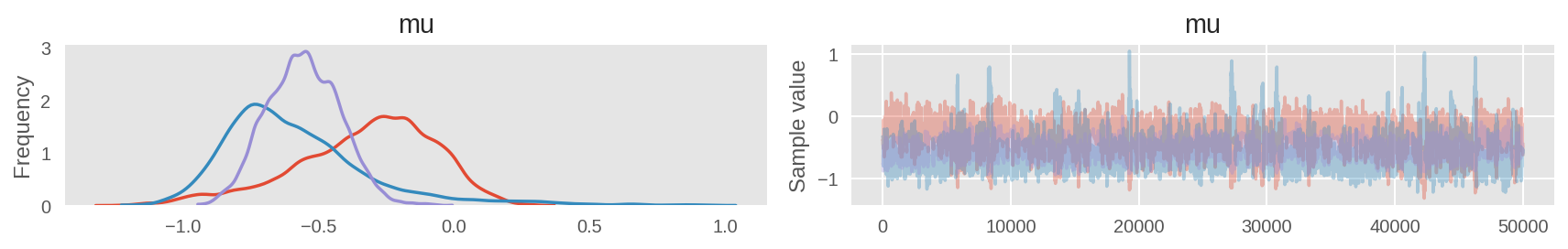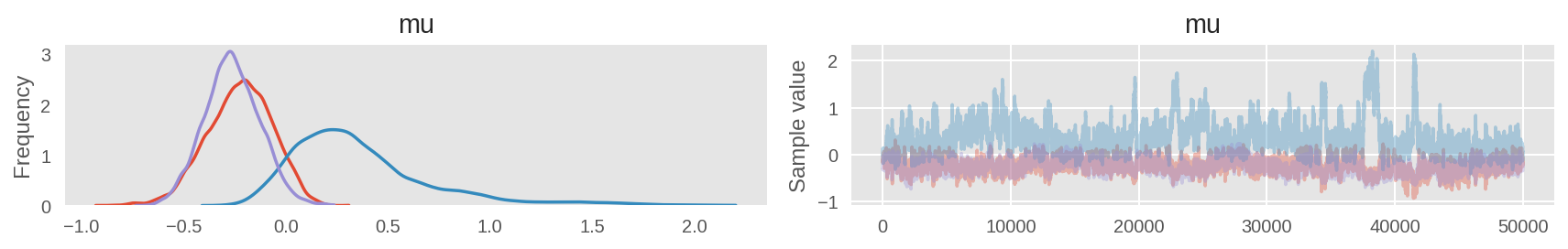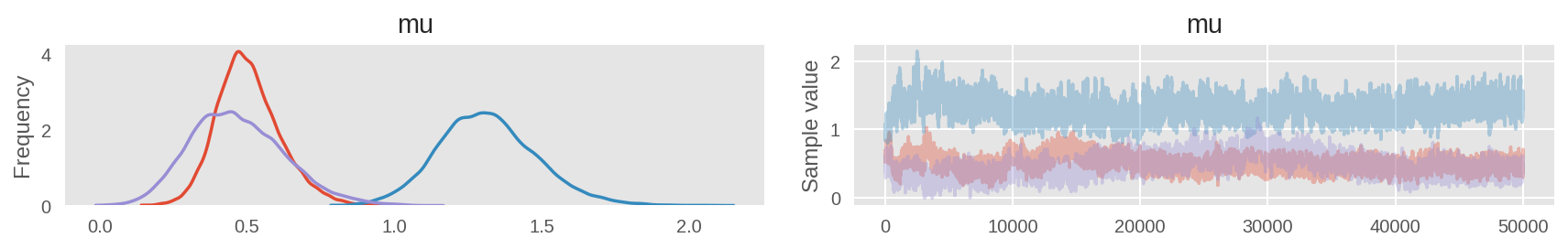In :
burnin=40000
import scipy.special as sp

fig = plt.figure(figsize=(15, 5))
gs = gridspec.GridSpec(1, 3)
x1=np.linspace(0, 1, 200)
plotparm=[r'$\mu_c$', r'$\mu_r$', r'$\mu_u$']
trialname=['Trial 1', 'Trial 2', 'Trial 6']
ax0 = {}

def npPhi(x):
# probit transform
return (1.0 + sp.erf(x / np.sqrt(2.0))) / 2.0

for trial in np.arange(Nt):
mupost=indiv_trial2[trial]['mu'][burnin:]
for ip, ii in enumerate(plotparm):
cpost = npPhi(mupost[:, ip])
ax0[ip] = plt.subplot(gs[ip])
my_pdf = gaussian_kde(cpost)
ax0[ip].plot(x1, my_pdf(x1), lw=2.5, alpha=0.6) # distribution function
plt.xlim([0, 1])
plt.xlabel(ii, fontsize=15)

ax0 = plt.subplot(gs)
plt.legend(trialname, loc='upper left')
plt.ylabel('Probability Density')
plt.show()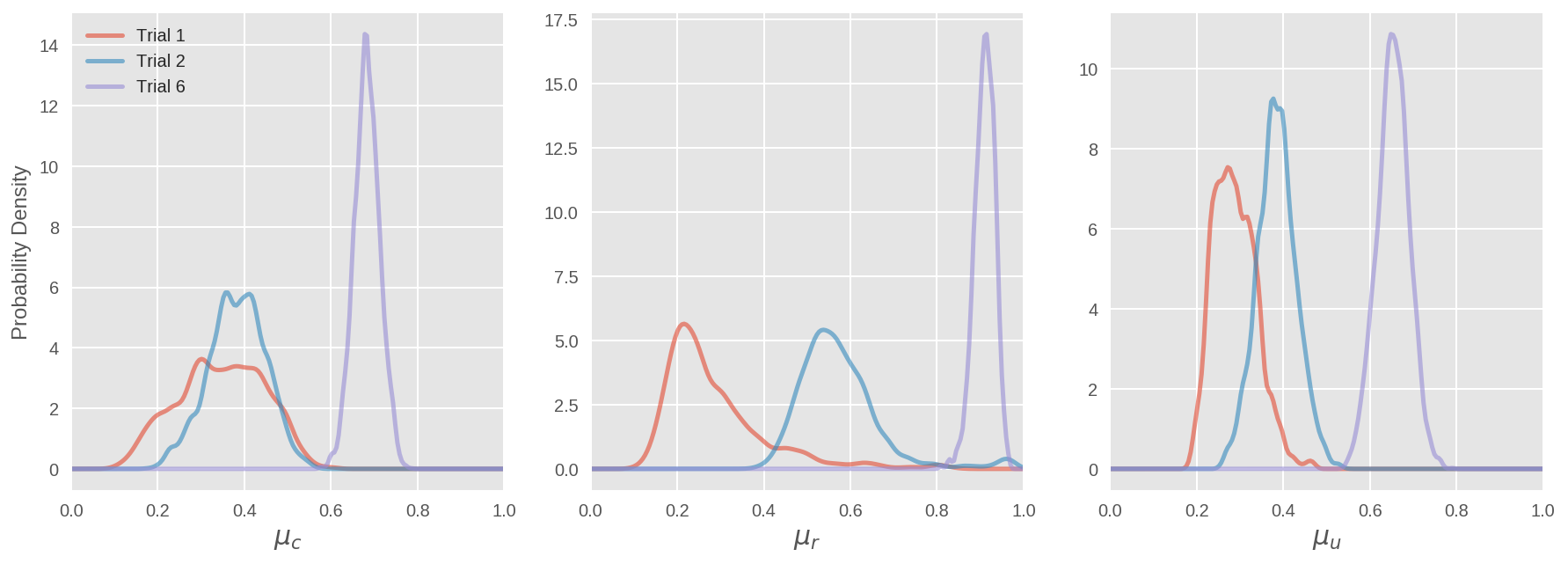In :
fig = plt.figure(figsize=(15, 5))
gs = gridspec.GridSpec(1, 3)
x1=np.linspace(-1, 1, 200)
plotparm2=[r'$\rho_{cr}$', r'$\rho_{cu}$', r'$\rho_{ru}$']

for trial in np.arange(Nt):
sigmainvpost=indiv_trial2[trial]['wishart'][burnin:]
tmpn=sigmainvpost.shape
sigmapost=np.ndarray((tmpn, 3, 3), dtype=float)
for i in range(tmpn):
sigmapost[i, :, :]=np.linalg.inv(sigmainvpost[i, :, :])

cpost0 = sigmapost[:, 0, 1]/np.sqrt(sigmapost[:, 0, 0]*sigmapost[:, 1, 1])
ax0 = plt.subplot(gs)
my_pdf = gaussian_kde(cpost0)
ax0.plot(x1, my_pdf(x1), lw=2.5, alpha=0.6) # distribution function
plt.xlim([-1, 1])
plt.xlabel(plotparm2, fontsize=15)

cpost1 = sigmapost[:, 0, 2]/np.sqrt(sigmapost[:, 0, 0]*sigmapost[:, 2, 2])
ax1 = plt.subplot(gs)
my_pdf = gaussian_kde(cpost1)
ax1.plot(x1, my_pdf(x1), lw=2.5, alpha=0.6) # distribution function
plt.xlim([-1, 1])
plt.xlabel(plotparm2, fontsize=15)

cpost2 = sigmapost[:, 1, 2]/np.sqrt(sigmapost[:, 1, 1]*sigmapost[:, 2, 2])
ax2 = plt.subplot(gs)
my_pdf = gaussian_kde(cpost0)
ax2.plot(x1, my_pdf(x1), lw=2.5, alpha=0.6) # distribution function
plt.xlim([-1, 1])
plt.xlabel(plotparm2, fontsize=15)

ax0 = plt.subplot(gs)
plt.legend(trialname, loc='upper left')
plt.ylabel('Probability Density')
plt.show()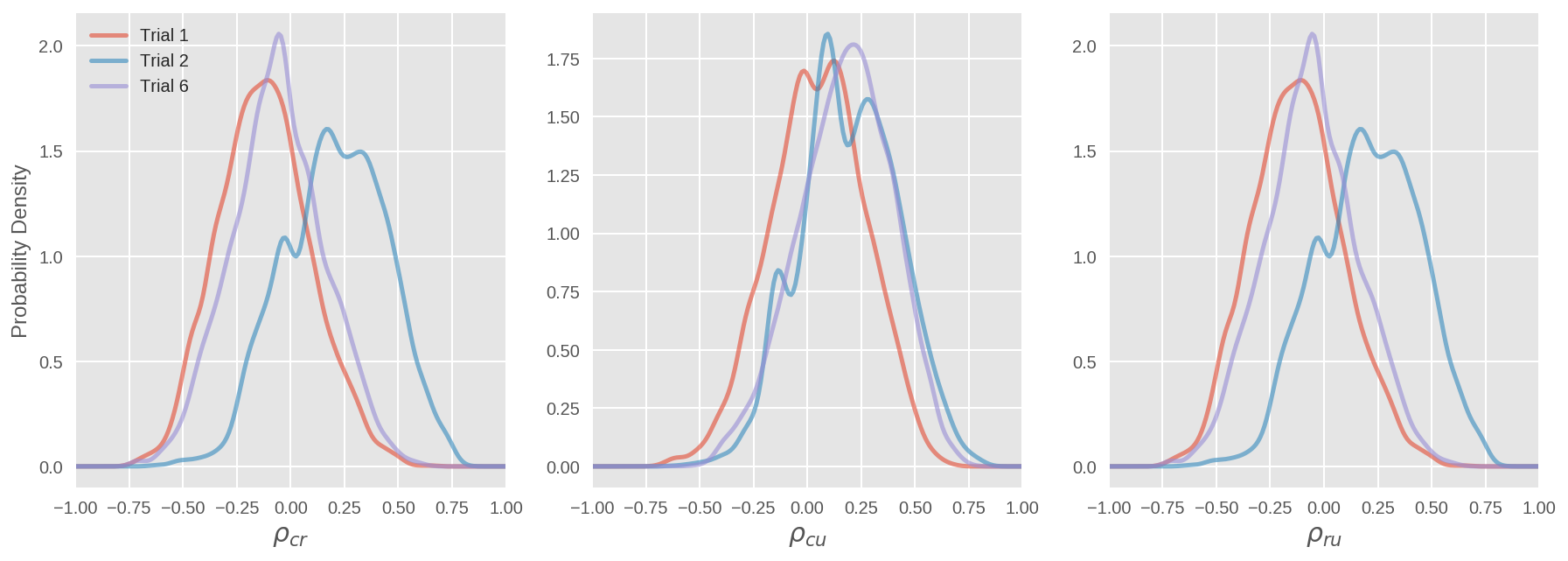The above model works fine, but using Wishart is highly discouraged, including the Wishart-Bartlett form. Below we reparameterized the model using a LKJ distribution (Cholesky decomposed version) as prior for covariance matrix.

In :
with pm.Model() as modelk:
mu = pm.Normal('mu', mu=0, sd=1, shape=Nt)
xi = pm.HalfNormal('xi', sd=10, shape=Nt)

sd_dist = pm.HalfCauchy.dist(beta=2.5)
packed_chol = pm.LKJCholeskyCov('chol_cov', n=Nt, eta=1, sd_dist=sd_dist)
# compute the covariance matrix
chol = pm.expand_packed_triangular(Nt, packed_chol, lower=True)

vals_raw = pm.Normal('vals_raw', mu=0, sd=1, shape=(Nt, Nsubj))
delta = tt.dot(chol, vals_raw).T

c = Phi(mu+xi*tt.dot(delta, [1, 0, 0]))
r = Phi(mu+xi*tt.dot(delta, [0, 1, 0]))
u = Phi(mu+xi*tt.dot(delta, [0, 0, 1]))

t1 = c*r
t2 = (1-c)*(u**2)
t3 = 2*u*(1-c)*(1-u)
t4 = c*(1-r)+(1-c)*(1-u)**2

muc = pm.Deterministic('muc', Phi(mu))
mur = pm.Deterministic('mur', Phi(mu))
muu = pm.Deterministic('muu', Phi(mu))

p_ = tt.transpose(tt.stack([t1, t2, t3, t4]))
kobs = pm.Multinomial('kobs', p=p_, n=Nitem, observed=k)
trace = pm.sample(3e3, njobs=2, init=None)

pm.traceplot(trace, varnames=['mu', 'xi']);

Assigned NUTS to mu
Assigned NUTS to xi_log__
Assigned NUTS to chol_cov_cholesky_cov_packed__
Assigned NUTS to vals_raw
100%|█████████▉| 3497/3500.0 [02:25<00:00, 27.51it/s]/home/laoj/Documents/Github/pymc3/pymc3/step_methods/hmc/nuts.py:467: UserWarning: Chain 0 contains 317 diverging samples after tuning. If increasing target_accept does not help try to reparameterize.
% (self._chain_id, n_diverging))
100%|██████████| 3500/3500.0 [02:25<00:00, 24.06it/s]
/home/laoj/Documents/Github/pymc3/pymc3/step_methods/hmc/nuts.py:451: UserWarning: The acceptance probability in chain 1 does not match the target. It is 0.577016461846, but should be close to 0.8. Try to increase the number of tuning steps.
% (self._chain_id, mean_accept, target_accept))
/home/laoj/Documents/Github/pymc3/pymc3/step_methods/hmc/nuts.py:467: UserWarning: Chain 1 contains 481 diverging samples after tuning. If increasing target_accept does not help try to reparameterize.
% (self._chain_id, n_diverging))